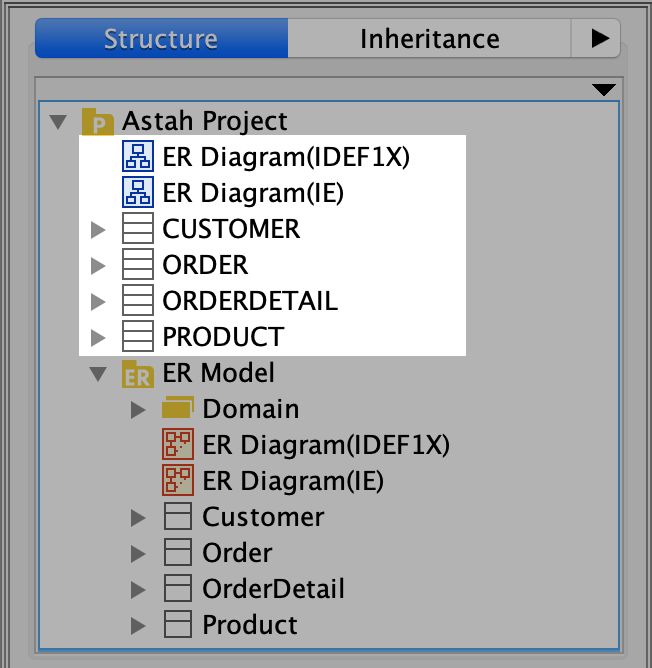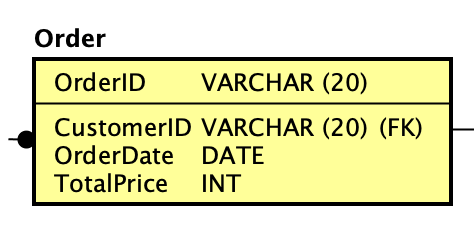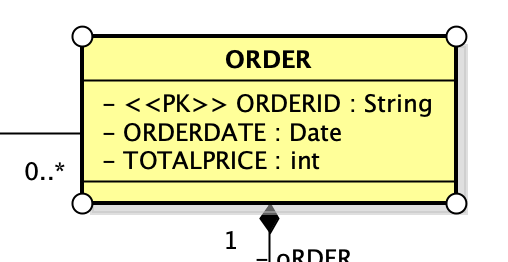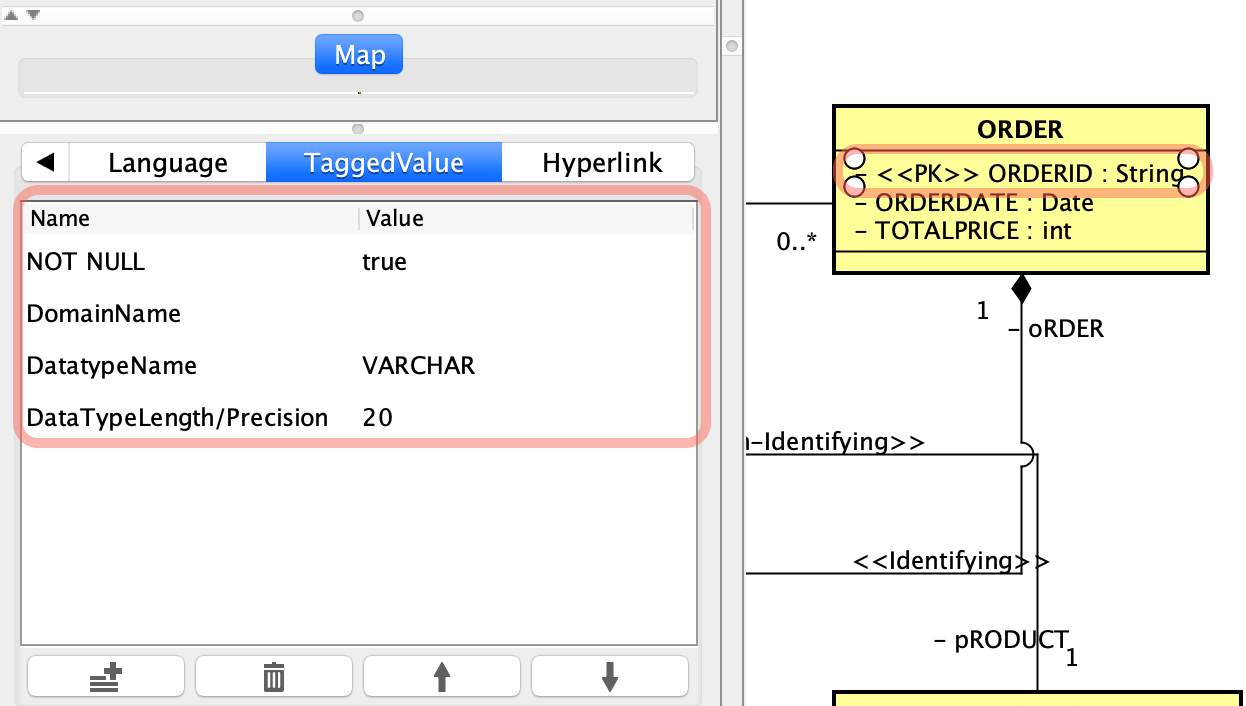In Astah Professional, you can convert ER diagrams to UML Class diagrams.## Convert ER diagram to Class diagram

1. Select [Convert to Class Diagram] from ER Diagram’s pop-up menu in the structure tree.2. The diagram and all the model elements inside will be converted to a Class diagram and appears in the tree view.## Convert ER models to UML models

In Astah Professional, instead of converting ER diagram to Class diagram one by one, you can convert multiple-diagrams and models all at once.

1. Go to [Tools] – [ER Diagrams] – [Convert ER Model to UML Model].2. Select which ER models you want to convert then click [Create].3. Selected models would be added in the tree view. To open the converted diagram, click [Open Diagram] menu in the tree view.## Convert Rule

All the ERD data type would be converted as below. Other data types will be shown as String on the diagram. However, the original data type information will be imported as “tagged value”. (Read More)

##### Convert to
INT
int
BIT
boolean
NUMERIC
int
CHAR
char or String (It will convert to char if its Length/Precision is none or 1.)
DATE
Date
DOUBLE_PRECISION
double
FLOAT
float## Data Type

When you convert ERD to UML, ER Entity’s data type will be exported as “Tagged Value” of Attributes in UML.

For example, there is an ER entity as below:When you convert this ER Entity, you will get a UML class like below:As you can see, VARCHAR (20) will be converted to String. However, the original data types are imported as “taggedvalue”. So when you open [TaggedValue] tab, you can see its original data type information.If you convert ERD to UML, then convert it back to ERD again, you can run this script using [Script Editor] to restore the original data type in the final ERD.